移除数组中的数字，不用额外空间, 实战 RxSwift 中的 Observable, subscribe, dispose, 吴军老师态度读后感 John 易筋 ARTS 打卡 Week 39John(易筋)

1. Algorithm: 每周至少做一个 LeetCode 的算法题

LeetCode 全集请参考：LeetCode Github 大全

# 题目

Given an array nums and a value val, remove all instances of that value in-place and return the new length.

Do not allocate extra space for another array, you must do this by modifying the input array in-place with O(1) extra memory.

The order of elements can be changed. It doesn't matter what you leave beyond the new length.

Clarification:

Confused why the returned value is an integer but your answer is an array?

Note that the input array is passed in by reference, which means a modification to the input array will be known to the caller as well.

Internally you can think of this:

// nums is passed in by reference. (i.e., without making a copy)int len = removeElement(nums, val);
// any modification to nums in your function would be known by the caller.// using the length returned by your function, it prints the first len elements.for (int i = 0; i < len; i++) { print(nums[i]);}

Example 1:

Input: nums = [3,2,2,3], val = 3Output: 2, nums = [2,2]Explanation: Your function should return length = 2, with the first two elements of nums being 2.It doesn't matter what you leave beyond the returned length. For example if you return 2 with nums = [2,2,3,3] or nums = [2,2,0,0], your answer will be accepted.

Example 2:

Input: nums = [0,1,2,2,3,0,4,2], val = 2Output: 5, nums = [0,1,4,0,3]Explanation: Your function should return length = 5, with the first five elements of nums containing 0, 1, 3, 0, and 4. Note that the order of those five elements can be arbitrary. It doesn't matter what values are set beyond the returned length.

Constraints:

0 <= nums.length <= 1000 <= nums[i] <= 500 <= val <= 100

1. 逐位 copy 解法

class Solution {    public int removeElement(int[] nums, int val) {        // check edge        if (nums == null || nums.length == 0) {            return 0;        }        int index = 0;        for(int i = 0; i < nums.length; i++) {            if (nums[i] != val) {                nums[index] = nums[i];                index++;            }        }                return index;    }}

2. 头尾想换 高效率解法

class Solution {    public int removeElement(int[] nums, int val) {        // check edge        if (nums == null || nums.length == 0) {            return 0;        }        int index = 0;        int n = nums.length - 1;        while (index <= n) {            if (nums[index] == val) {                nums[index] = nums[n];                nums[n] = val;                n--;            } else {                index++;            }        }                return index;    }}

可观察的生命周期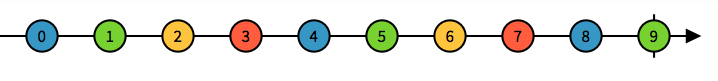Observable发出三个轻击事件，然后结束。这称为completed事件。

初始化环境

# Uncomment the next line to define a global platform for your project# platform :ios, '9.0'
target 'RXSwiftDemo' do # Comment the next line if you don't want to use dynamic frameworks use_frameworks!
# Pods for RXSwiftDemo pod 'RxSwift', '6.1.0' pod 'RxCocoa', '6.1.0'
target 'RXSwiftDemoUITests' do pod 'RxBlocking', '6.1.0' pod 'RxTest', '6.1.0' end
end

创建 observable

just恰当地命名，因为它所做的只是创建一个包含just单个元素的可观察序列。

let one = 1  let two = 2  let three = 3  /// This is a singe element observable  let observable: Observable<Int> = Observable<Int>.just(one)    /// This is an observable of individual type instances from a array  let observable2 = Observable.of(one, two, three)    /// This is an observables array using of to create  let observable3 = Observable.of([one, two, three])    /// This is an observable of individual type instances from a array  let observable4 = Observable.from([one, two, three])

订阅观察值 subscribe

let one = 1  let two = 2  let three = 3  /// This is an observable of individual type instances from a array  let observable = Observable.of(one, two, three)    /// Subscribes an element handler  observable.subscribe(onNext: { element in    print(element) // Result will be: 1,2,3  })    /// Subscribes an event handler to an observable sequence.  observable.subscribe { event in    print(event) // Result will be: next(1) next(2) next(3) completed  }

empty操作者创建具有零个元素的空可观察序列。它只会发出一个.completed事件。

let observable = Observable<Void>.empty()    observable      .subscribe(        onNext: { element in          print(element)      },        /// When empty an observable, it will go to completed block        onCompleted: {          print("Completed")      }    )

never操作创建一个可观察到的不排放任何东西，从来没有终止

/// If never an observable, it will not emit anythinglet observable = Observable<Any>.never()    observable      .subscribe(        onNext: { element in          print(element)      },        onCompleted: {          print("Completed")      }    )

处置和终止 dispose

let observable = Observable.of("A", "B", "C")  let subscription = observable.subscribe { event in    print(event)  }  /// When dispose, the observable can not emit anything  subscription.dispose()

1. 什么是状态图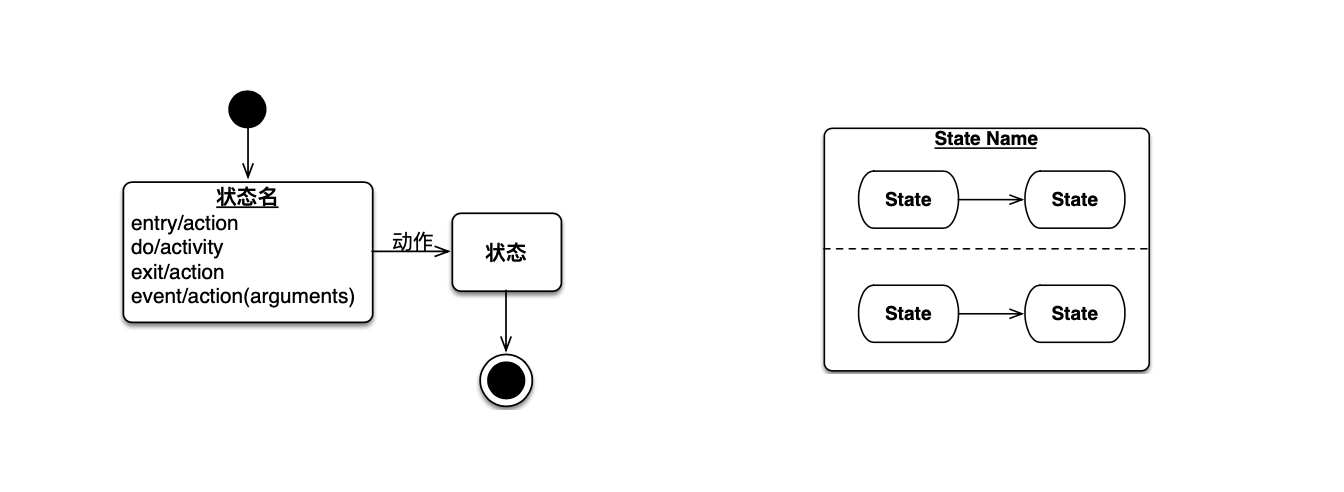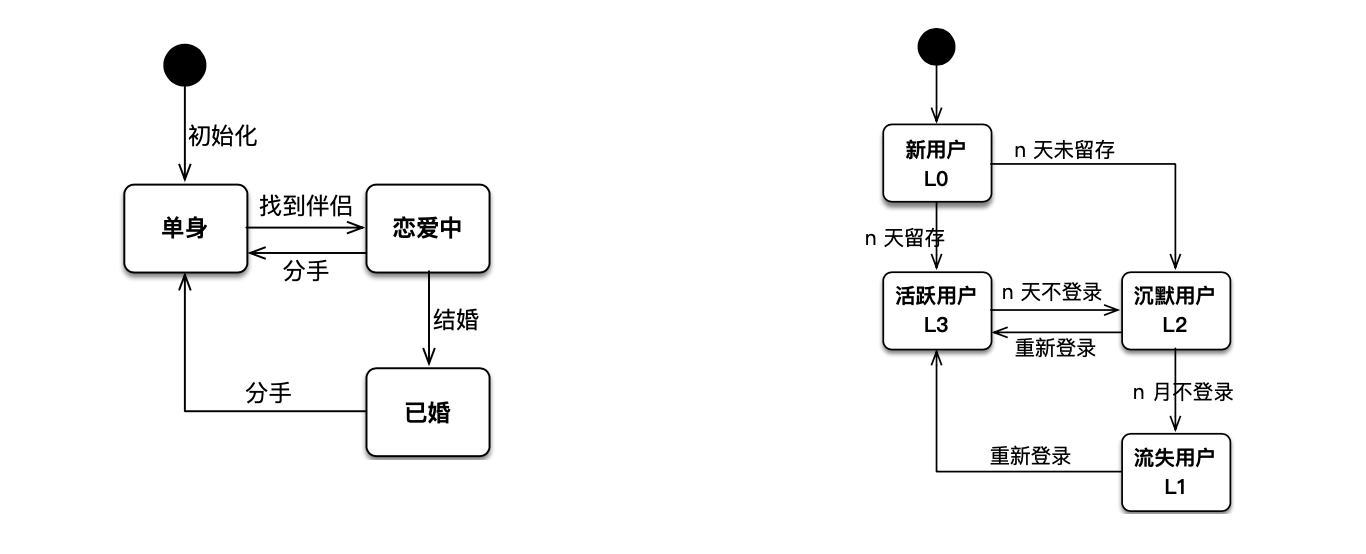2. 动手画 -- 员工状态转换状态图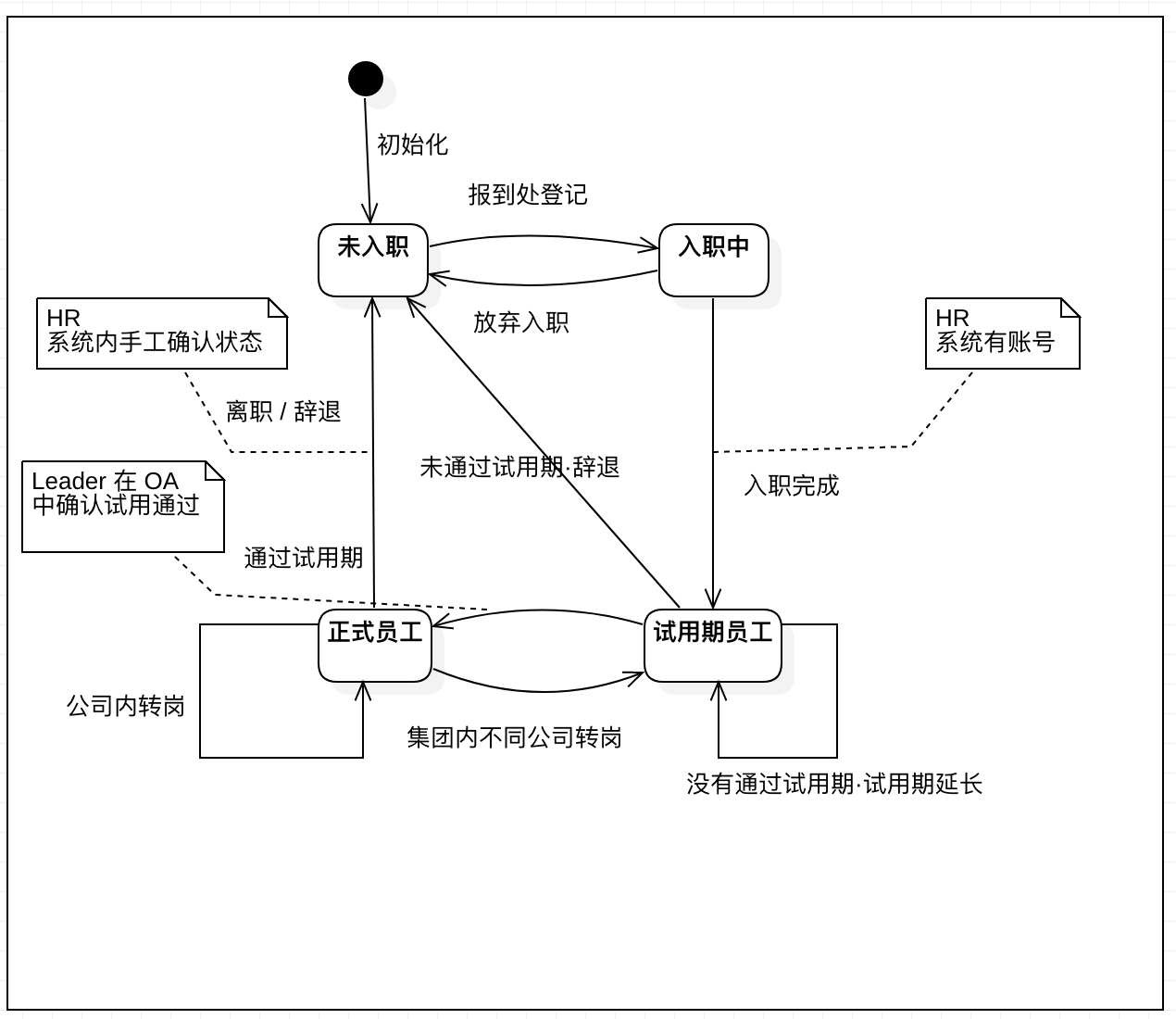1. 加个白底的背景框；

2. 注释要用 45°的折角；

3. 状态相互转换用曲线。

4. 什么是概念模型

Kindle，阅读类软件的概念模型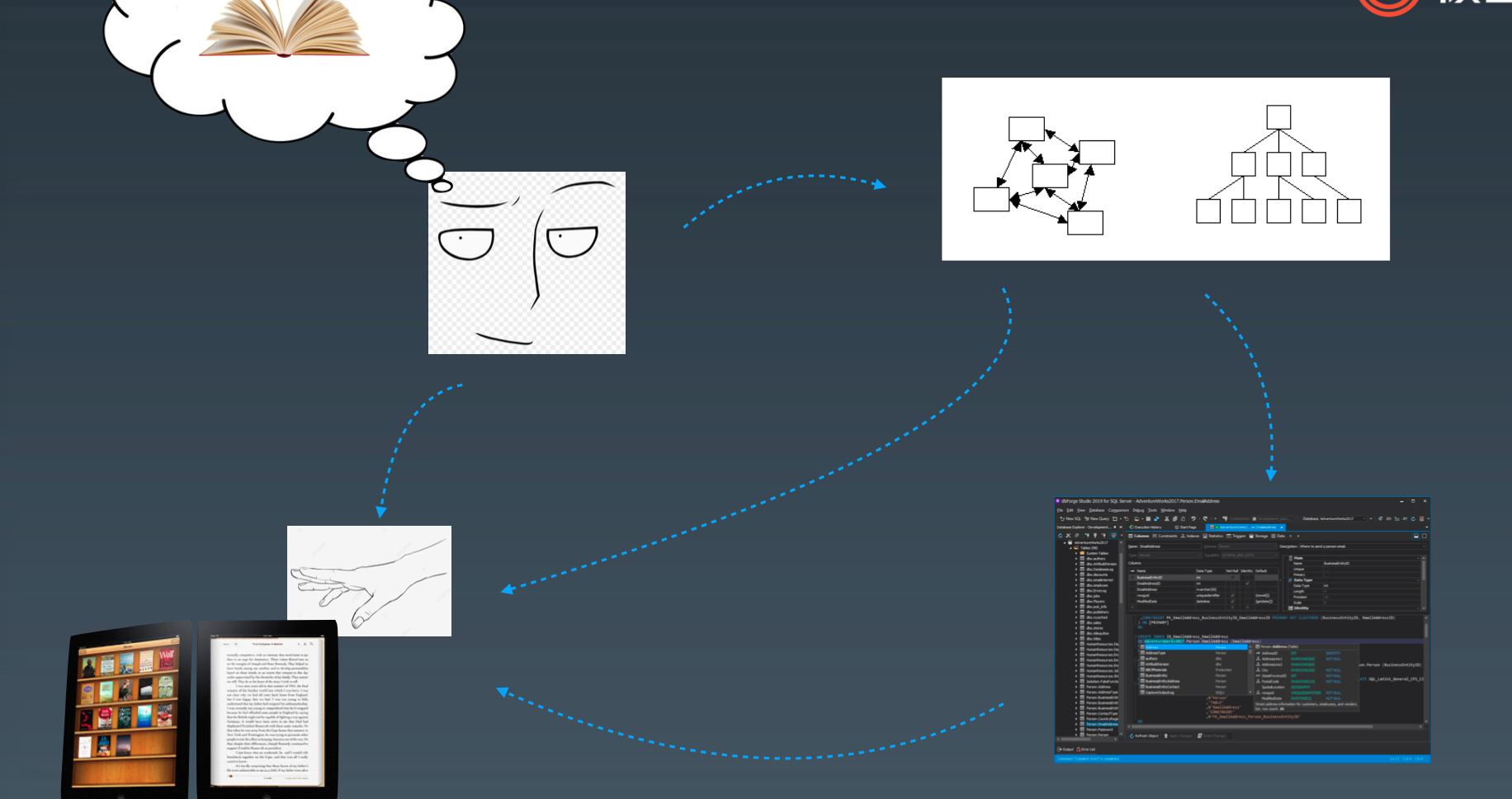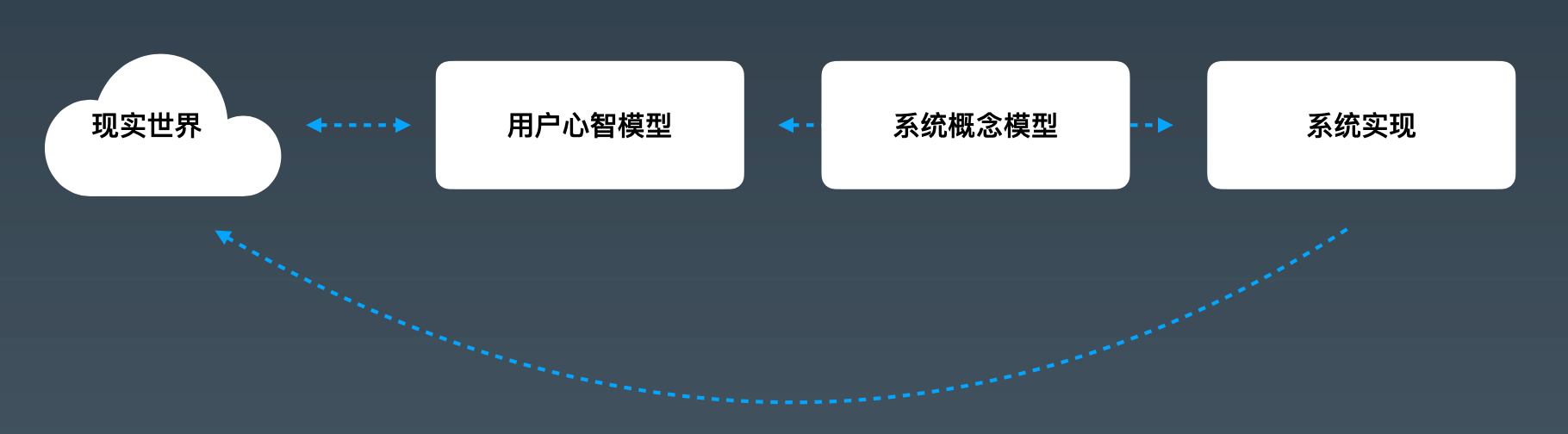5. 心智模型6. 概念模型

• 在现实世界中，是一段描述

• 在程序世界中，是代码和数据结构

• 在用户眼里，是一堆样式、交互和界面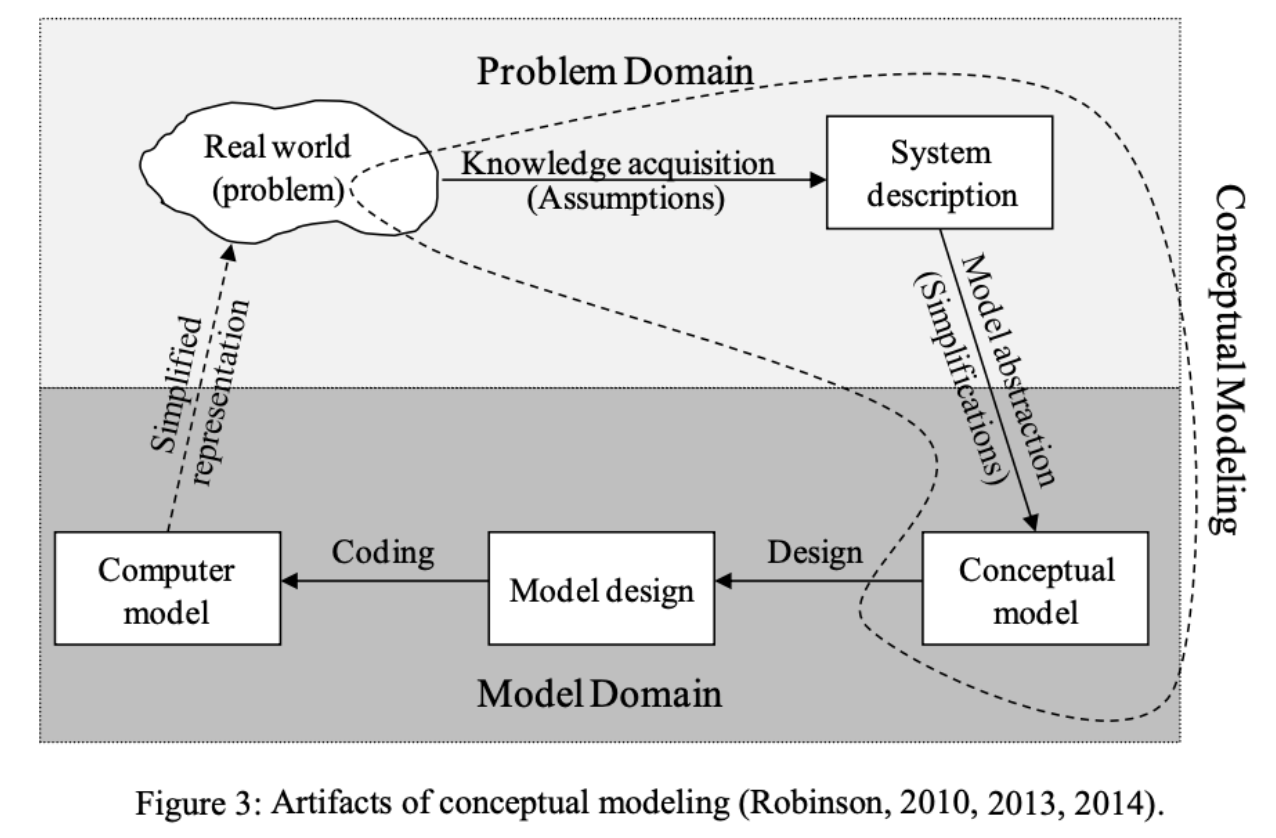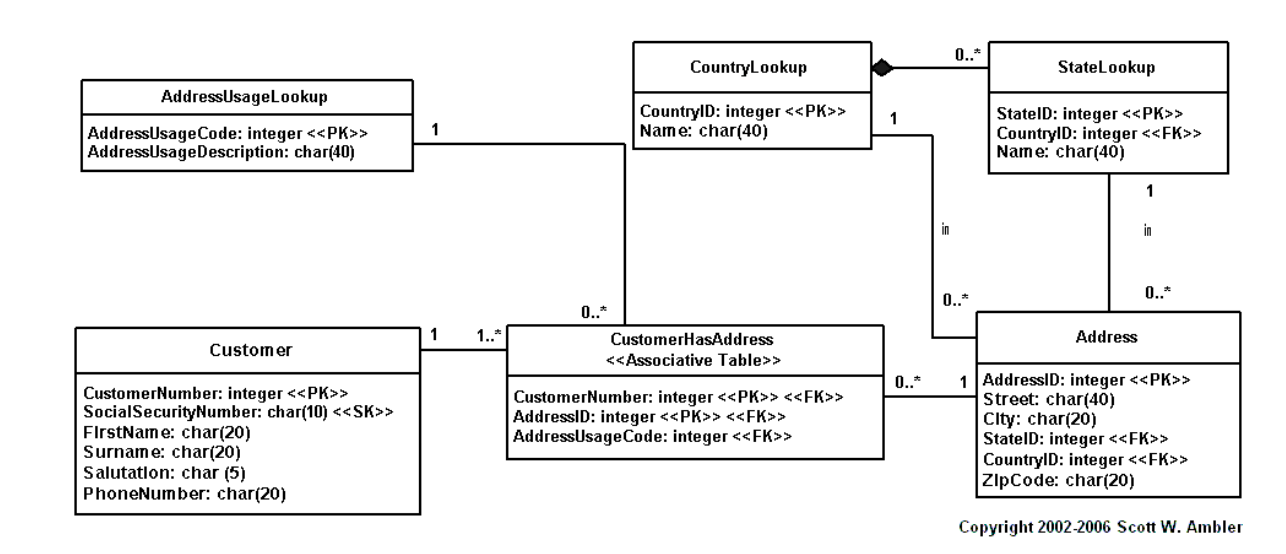7. 概念模型设计过程

• 用领域语言和用户语言进行【描述】，名词是可能的【实体】，动词是可能的【关系】。

• 在不同的名字空间中，统一术语，删减不必要的【实体】。

• 定义每两者之间的关系，并明确【一对多】，【多对一】，【一对一】，【多对多】的原则。

• 找到不同实体的行为。

8. 动手画 -- 微信视频号 概念模型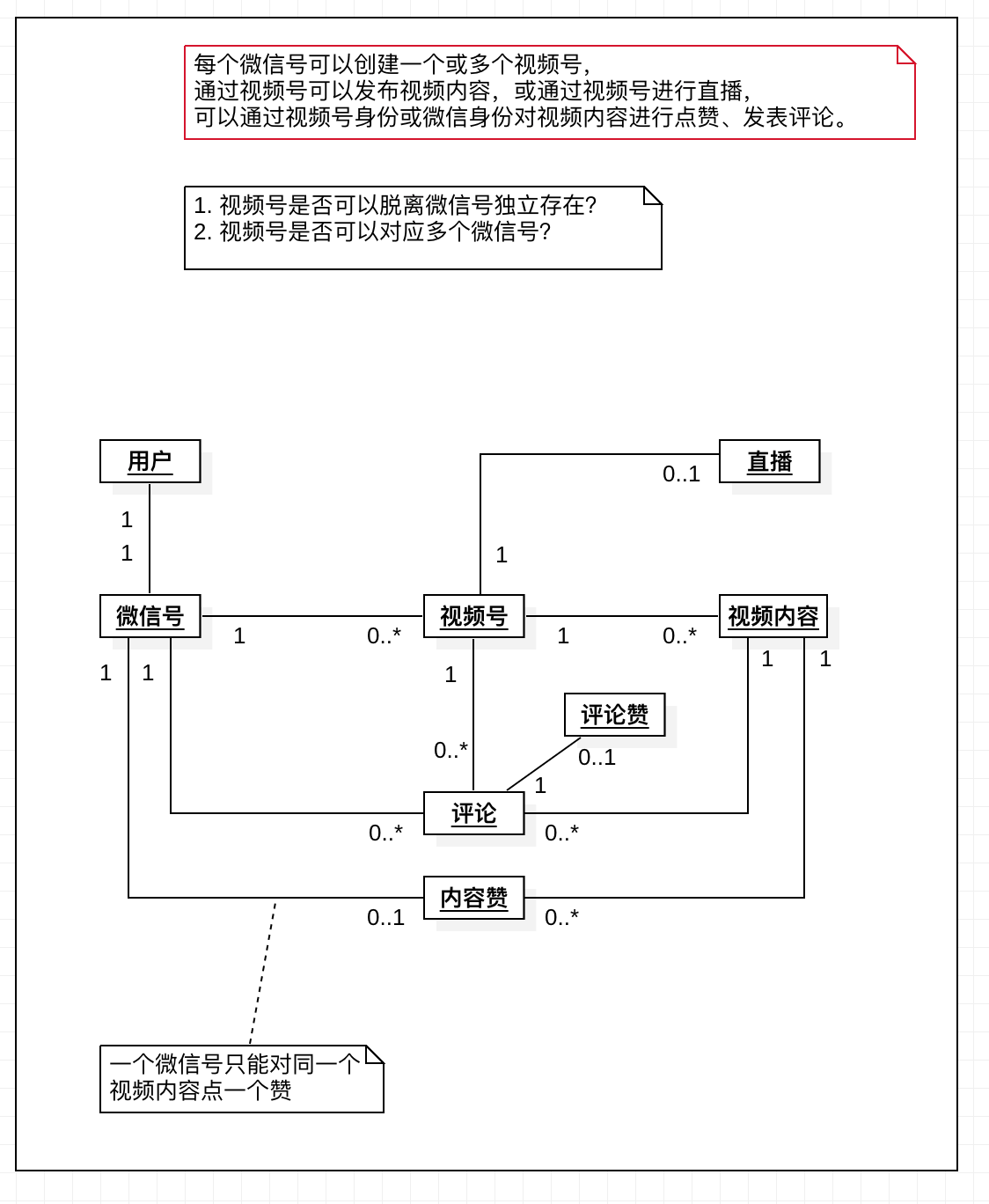9. 概念模型的价值

• 有一个【完整】的系统视角，看到系统内的概念以及关系。

• 帮助思考和澄清一些边界性的问题。

• 有一个明确的概念关系统一沟通，统一开发、业务和用户视角。

9.1 概念模型的风险和问题

• 画图一时爽，读图有可能比较困难。

• 要靠大量的沟通，很难独立读懂。

• 模型和当前用例/业务规则之间，可能存在差异（比如一个微信号关联几个视频号？）

9.2 一点小小的经验

• 概念模型图，很多时候，对自己的意义大于对外沟通，是一个结构化提问的过程。

• 通常做过开发，设计过表结构的人，更容易理解这个东西是啥，建议学。

• 可能是需要迭代更多的一种图。

• To B 业务中通常更加需要。

• 规模到一定程度，可能就很难读懂了，建议有机拆解。

说明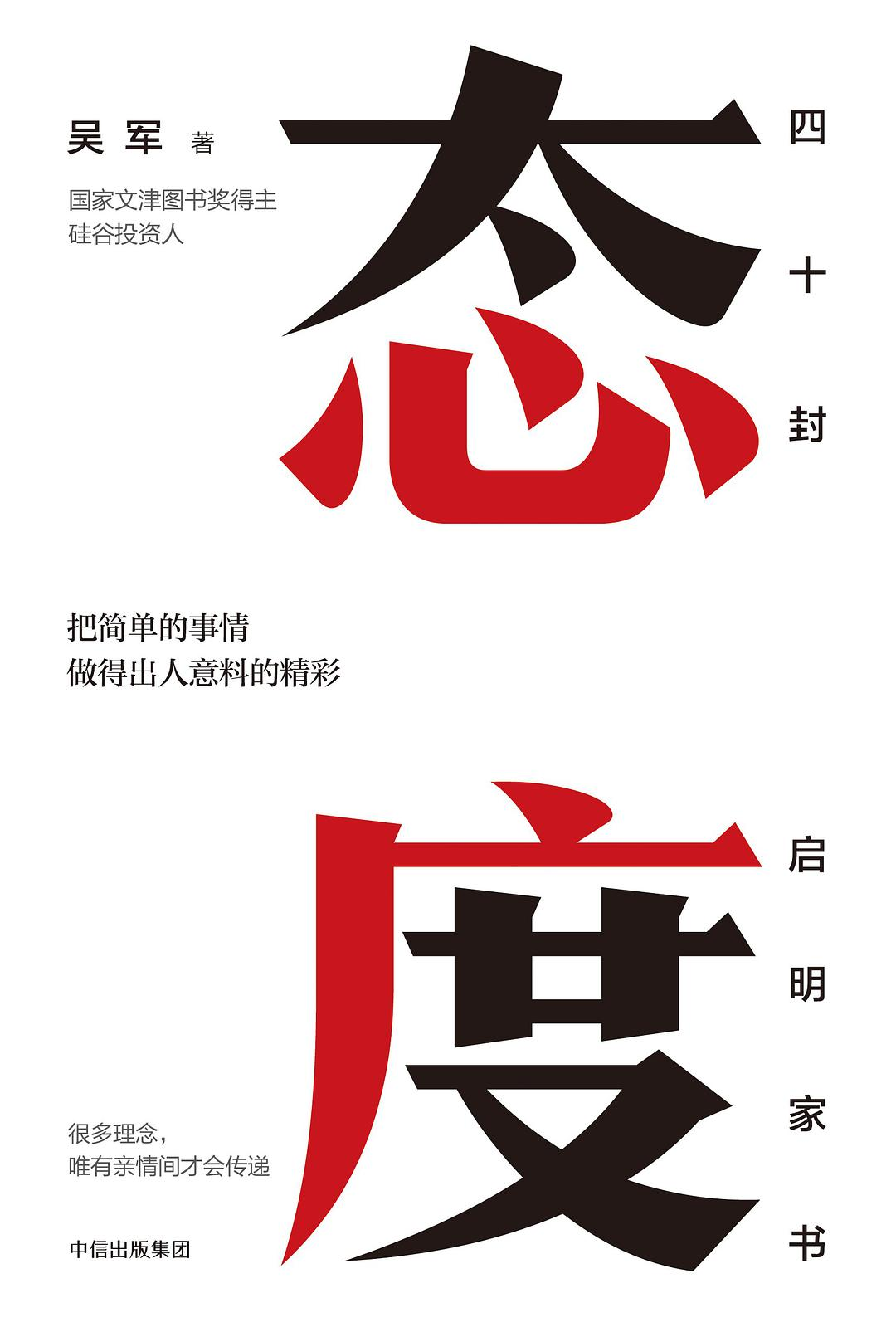《态度》- 吴军 四十封启明家书 读后感。此书相当大的内容和得到的《硅谷来信一》、《硅谷来信二》、《硅谷来信三》专栏重复。闪光点在于以家书的形式，吴军跟两位女儿梦华、梦馨的书信对话。

1. 人生哲学

1.1 乐观的人生态度

1. 不断地接收教育，与时俱进。

2. 有理想并努力实现自己的愿望。

3. 与人相处共事，尽可能互相尊重，相互包容。

4. 看透人生。

1.2 幸福的来源

1. 幸福的来源来自基因的传承。吴军曾经说过人生必须做的第一件事是恋爱、结婚、生子。

2. 人生的一条河，人的影响力有河流的长度、深度、广度三个维度决定的。要有所传承。

1.4 好习惯成就一生

1. 注意你的想法，因为它能决定你的言辞和行动。

2. 注意你的言辞和行动，因为它能主导你的行为。

3. 注意你的行为，因为它能变成你的习惯。

4. 注意你的习惯，因为它能塑造你的性格。

5. 注意你的性格，因为它能决定你的命运。

2 洞察世界

2.1 好运气背后是三倍的努力

>为什么呢？我是这样想的，当许多聪明、求知欲强、富有同情心且目光敏锐的年轻人聚到一起，即使没有人教，他们也会相互学习。他们会互相交流，了解新的思想和看法，看到新鲜的事物，并且掌握独到的行为判断力。

6. 做人做事评论